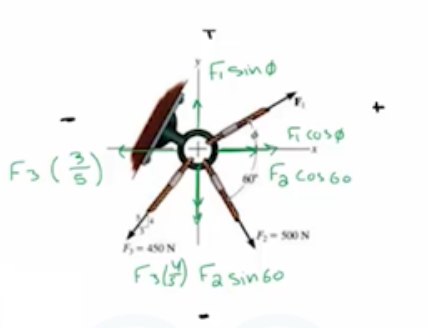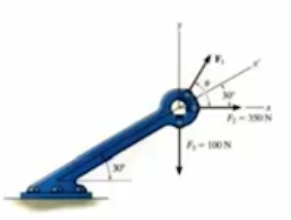Need Help?

Subscribe to Statics

###### \${selected_topic_name}
• Notes
• Comments & Questions

If $F_{1}=600 \mathrm{N}$ and $\phi=30^{\circ}$, determine themagnitude of the resultant force acting on the eyebolt and its direction measured clockwise from the positive $x$ axis.

x- Direction 9-

$F_{1} \cos \phi+F_{2} \cos 60-F_{3}\left(\frac{3}{5}\right)$

$= 600 \cos 30+500 \cos 60-450\left(\frac{3}{5}\right)=(499.6 \mathrm{N})$y- Direction 9-

$F_{1} \sin \phi-F_{2} \sin 60-F_{3}\left(\frac{4}{5}\right)=$

$=600 \sin 30-500 \sin 60-450\left(\frac{4}{5}\right)=-493 N=(493 N)$

$∴ F_{R}=\sqrt{(499.6)^{2}+(493)^{2}}=702 N$

$∴ \tan \theta=\frac{493}{499 \cdot 6} \rightarrow \theta=44 \cdot 6^{\circ}$

Express each of the three forces acting on the bracket in Cartesian vector form with respect to the  $x$ and $y$ axes. Determine the magnitude and direction $\theta$ of $\mathbf{F}_{1}$ so thatthe resultant force is directed along the positive $x^{\prime}$ axis and has a magnitude of $F_{R}=600 \mathrm{N}$.$F_{1}=\left(\underbrace{F_{1} \cos \theta}_{x} i+\underbrace{F_{1} \sin \theta}_{y} j\right) N$

$F_{2}=350 i N$   $(350 i+0 j) N$

$F_{3}=\left(\begin{array}{lll}{0 i} & {-} & {100 j}\end{array}\right) N$

$+\rightarrow$ $\sum F_{x}=F_{R} \cos 30 \longrightarrow$$F_{1} \cos \theta \pm 350=600 \cos 30$

$\longrightarrow F_{1} \cos \theta=169.6 \longrightarrow (1)$

$+\uparrow \sum F_{y}=F_{R} \sin 30 \longrightarrow F_{1} \sin \theta=100=600 \sin 30$

$\rightarrow F_{1} \sin \theta=400 \longrightarrow (2)$

$∴ \tan \theta = \frac{400}{169 \cdot 6}=\theta=67^{\circ} \rightarrow \theta=670$

$∴ F_{1}=434 N$

No comments yet